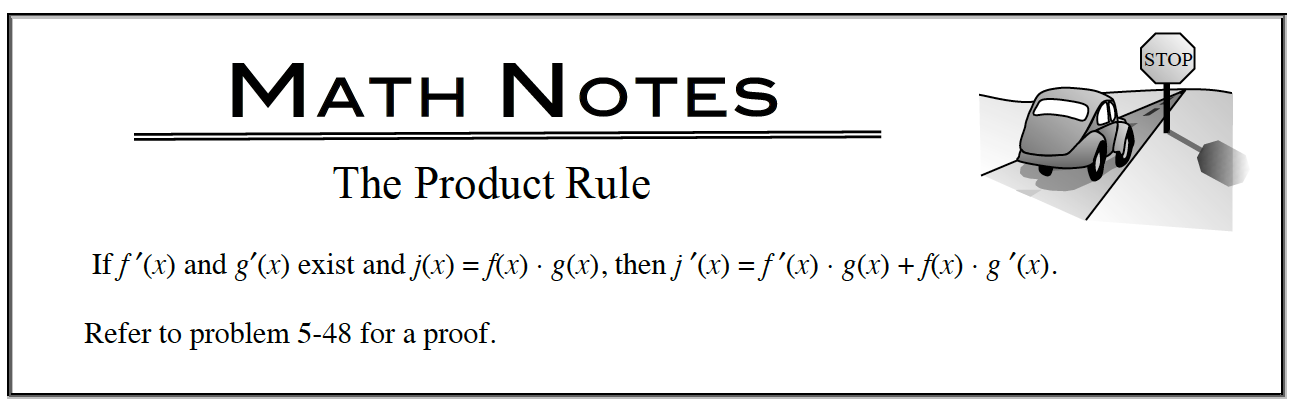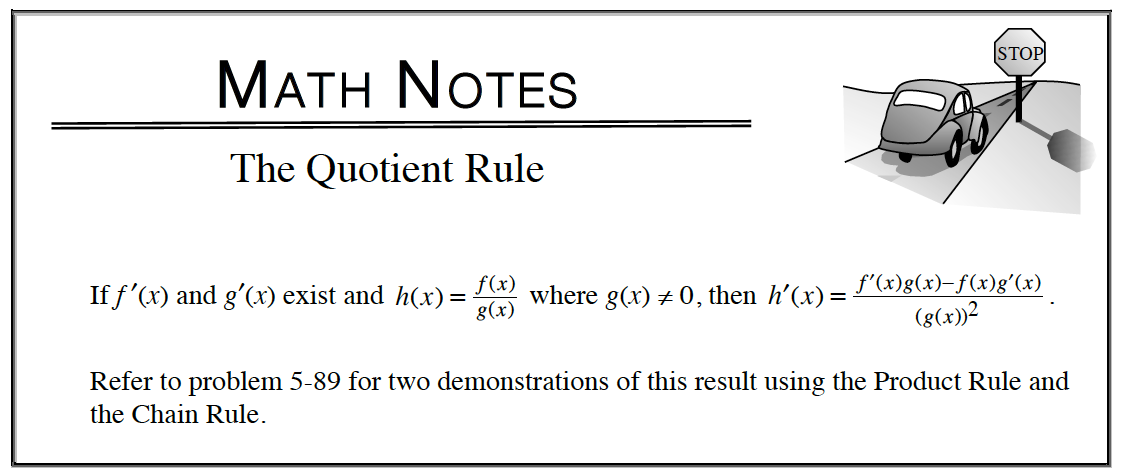### Home > APCALC > Chapter 6 > Lesson 6.1.2 > Problem6-26

6-26.

Examine the following derivatives. Consider the multiple tools available for differentiation and use the best strategy for each part. Evaluate each derivative and briefly describe your method. Homework Help ✎

1. $\frac { d } { d x } [ \operatorname { sin } ( x ) \cdot e ^ { x } ]$2. $\frac { d } { d z } [ \frac { 6 z + 1 } { 3 z - 2 } ]$3. $\frac { d } { d t } [ \operatorname { tan } ( t ) \cdot \operatorname { cos } ( t ) ]$

$\text{tan}(t)=\frac{\text{sin}(t)}{\text{cos}(t)}$